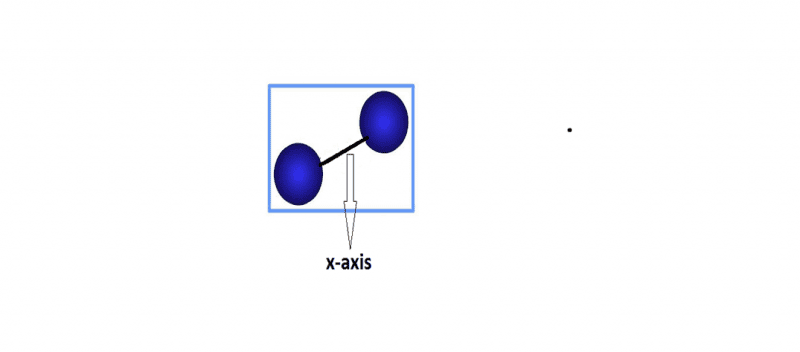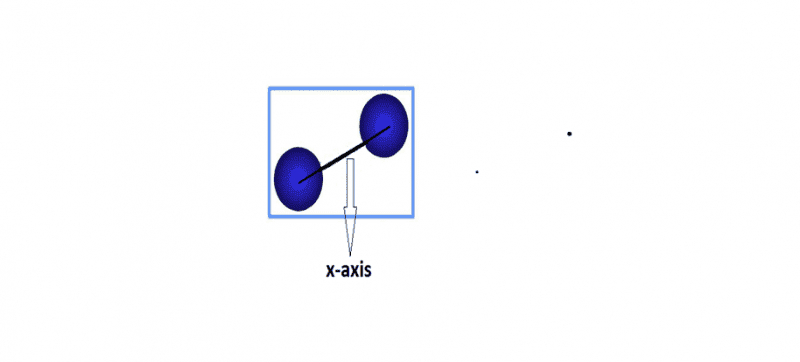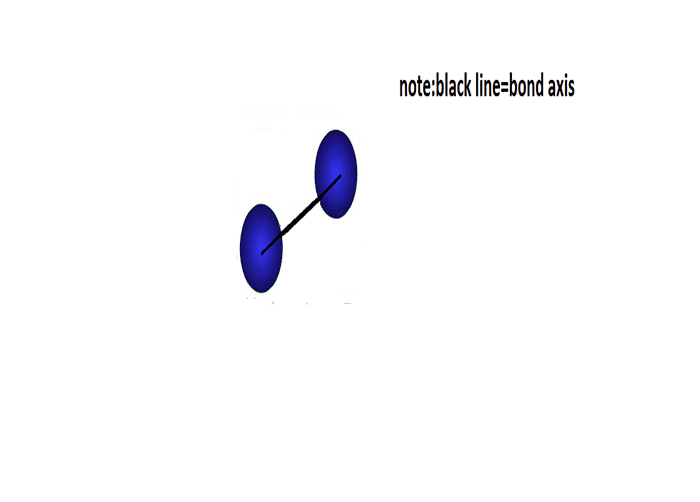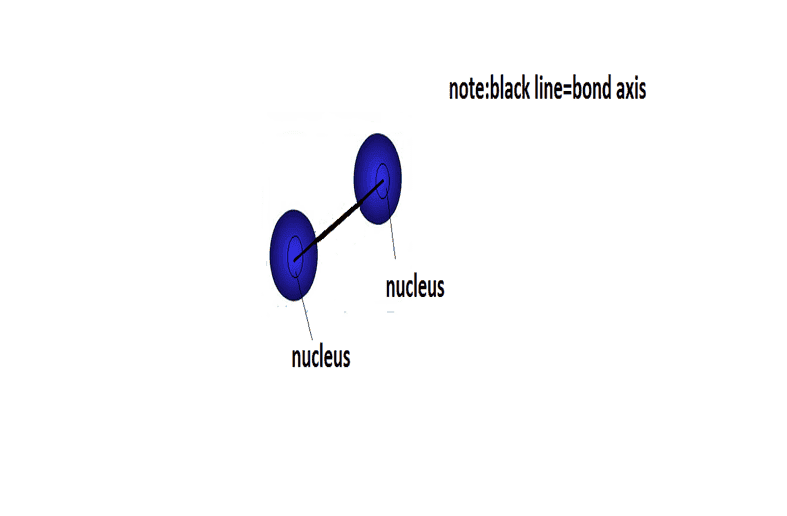# Moment of inertia

Why the moment of inertia of a molecule about the bond axis is small enough to ignore ?

Simon Bridge
Homework Helper
Try calculating it and see.

Try calculating it and see.
I really don't have any idea.Please guide me.

Bystander
Homework Helper
Gold Member
Where is the mass of an atom located?

•gracy
Where is the mass of an atom located?
throughout the atom.But being more technical i think where center of mass is present.

Simon Bridge
Homework Helper
How do you normally calculate the moment of inertia?
What would the distribution of mass in a diatomic molecule look like - roughly?

Bystander
Homework Helper
Gold Member
throughout the atom
"????" Back up. Describe the structure of an atom.

•gracy
"????" Back up. Describe the structure of an atom.
sorry i think the nucleus contain all mass as electrons have negligible mass.

Bystander
Homework Helper
Gold Member
Better. What's the diameter of a typical nucleus, or has that been covered for you at all?

•gracy
How do you normally calculate the moment of inertia?
What would the distribution of mass in a diatomic molecule look like - roughly?
I normally calculate moment of inertia as total mass multiplied by distance square.

Better. What's the diameter of a typical nucleus, or has that been covered for you at all?

Bystander
Homework Helper
Gold Member
Sounds about right. What's nuclear mass x 1/2 of that squared?

•gracy
What's nuclear mass x 1/2 of that squared?
Sorry I didn't understand.Nuclear mass would depend on atom of which element we are taking.
1/2 of that squared?
squared of what?

I normally calculate moment of inertia as total mass multiplied by Distance between the axis and rotation in m..

Bystander
Homework Helper
Gold Member
Sorry I didn't understand.Nuclear mass would depend on atom of which element we are taking.
Pick an atom.

squared of what?
"What?" The nuclear diameter. That's an end point of a bond axis.

•gracy
That's an end point of a bond axis.
Sorry i am talking about a molecule.Here x axis is bond axis.Bystander
Homework Helper
Gold Member
No, that is one of three rotational axes directed at right angles to one another through the center of mass of the molecule; the bond axis is the line connecting the nuclei of the two atoms, and is the one axis of the three that you're interested in for this problem.

•gracy
No, that is one of three rotational axes directed at right angles to one another through the center of mass of the molecule; the bond axis is the line connecting the nuclei of the two atoms, and is the one axis of the three that you're interested in for this problem.
Bystander is correct, the axis you have as your x-axis is one with a non-negligible moment of inertia. There would also be one perpendicular to this and the axis along the bond, which also has a non-negligible moment of inertia, this is why diatomic gases have two rotational degrees of freedom. The bond axis is that which joins the two nuclei.

[QUOTE="Bystander, post: 4940685, member: 50"the bond axis is the line connecting the nuclei of the two atoms,Now is x axis bond axis?

[QUOTE="Bystander, post: 4940685, member: 50"the bond axis is the line connecting the nuclei of the two atoms,
View attachment 76453
Now is x axis bond axis?
No, your black line (the bond) is (lies along) the bond axis.

No, your black line (the bond) is (lies along) the bond axis.
That's what i mean. Is black line bond axis?

That's what i mean. Is black line bond axis?
The black line joining the two blue atoms is the bond axis. Your diagram is confusing as the arrow suggests your x-axis is vertical and perpendicular to the bond.

The black line joining the two blue atoms is the bond axis. Your diagram is confusing as the arrow suggests your x-axis is vertical and perpendicular to the bond.Right?

That's better.
Now how to proceed further in order to get the answer .

Bystander
Homework Helper
Gold Member
What's the maximum distance of the nuclear mass from that axis?

•gracy
What's the maximum distance of the nuclear mass from that axis?
here (in the image i have given)there are two atoms( after all i am referring to diatomic molecule)nuclear mass of which one?

Bystander
Homework Helper
Gold Member
Both of them. How far are they from the axis you've drawn?

Both of them. How far are they from the axis you've drawn?I think answer would be ~nearly zero.

Bystander
Homework Helper
Gold Member
Yes. This is the point of asking you what nuclear dimensions are; so, calculating moments of inertia for the three rotational axes of a molecule about its center of mass, you have two that square something of the same order of magnitude as the bond length (not half in general, because not all diatomic molecules are homonuclear), and a third moment that squares a distance the order of nuclear dimensions.

•gracy
This is the point of asking you what nuclear dimensions are; so, calculating moments of inertia for the three rotational axes of a molecule about its center of mass, you have two that square something of the same order of magnitude as the bond length (not half in general, because not all diatomic molecules are homonuclear), and a third moment that squares a distance the order of nuclear dimensions.
Sorry but i am not getting this post.

Bystander
Homework Helper
Gold Member
Three questions: what size are bond lengths? What is typical nuclear diameter (radius)? What is the ratio of the squares of those two dimensions?

•gracy
bond length is the average distance between nuclei of two bonded atoms in a molecule. why are you asking for bond lengths?as there is only one ,right?[/QUOTE]
and why you have written radius inside the bracket after word diameter as these two are totally different.

Bystander
•